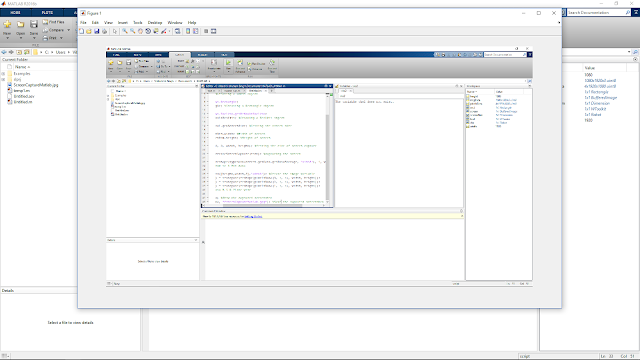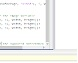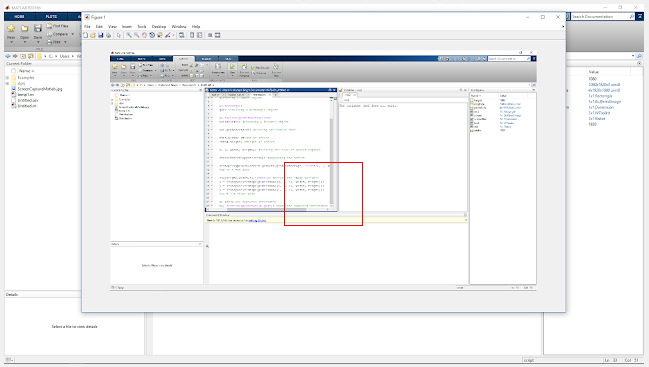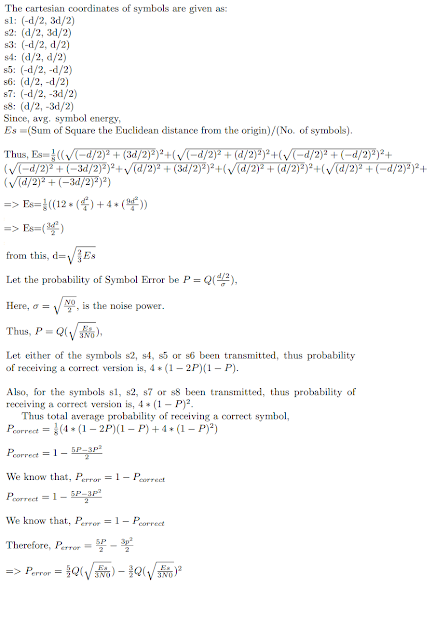# Template Matching in Grayscale/RGB image through Normalized Phase Correlation in MATLAB

In image processing, Template Matching is a technique to match a smaller portion of an image with a template image. Or in other words, a technique to find areas in the larger image that match a smaller (template) image.

So why template matching is generally used? For example in a product assembly line, if some of the product after visibly scanning is not matching with a good sample (template of a good sample product) then it could be automatically discarded.  An example is above.

Thus whenever our application needs to match a portion of the image with a reference image to judge its quality/location in a bigger image we need template matching.

Why phase correlation for template matching?
This method is generally fast, accurate, resilient to noise and can withstand the rotation as well as translation of the template within the image!

In our experiment: following is the image in which we will be searching for the template. The following code although tested for a grayscale image but it can work well even for a true-color image as well.Image to match with template (save it as image.jpg for using it in the code)

Template Image:Template image (save it as template.jpg for using it in the code)

MATLAB Code for Template Matching in Grayscale/RGB image through Normalized Phase Correlation
% comment in Bold
% This code provides 2 options to template match i.e., in frequency domain
% through normalized phase correlation. Normxcorr2 is included to act as a benchmark
% for speed and accuracy for both these methods. At the end of the code the
% execution time of processing is also displayed

%% Initial Console/Memory cleaning operations

clc
clear
close all
warning off

%% Options
disp(' Frequency Domain Processing through Normalized Phase Correlation- Can withstand tonal mismatch, translation, occlusion)(Fastest)');
disp(' Normalized Correlation (Matlab Function)- To act as a benchmark for execution time and accuracy ) (Meduium fast)');
option = input('Enter option[1 or 2]:');

%if images in working directory: give just full image name with extension
%if not in the working directory: provide the full path of the image file.

%image which is to be placed on the parent image
% image of whose pattern to be matched on the parent image
% uncomment below if wanted to extract an image portion from the original image to create a new matching template.
% im2match_orig = imcrop(im2process_orig, [100 250 size(im2paste,1)-1 size(im2paste,2)-1]);

if(size(im2process_orig,1)<size(im2match_orig,1)||size(im2process_orig,1)<size(im2match_orig,1))
disp('The size of the template should be smaller than the image! Please try again after correcting this!');
return;
end
%checking for precondition (size of template < size of image)

offset_row=0;
offset_col=0;
%above are the coordinates correction offset introduced by the method

subplot(3,3,[1,2,3,4,5,6])
imshow(im2process_orig); title('Parent image')
hold on
subplot(3,3,7)
imshow(im2match_orig); title('Image pattern to find')
disp('Step 1/5 completed successfully!')
%% Ensuring we get a grayscale image to process

if(size(im2process_orig,3)>1)
im2process    = rgb2gray(im2process_orig);
else
im2process    = im2process_orig;
end
%rgb2gray works as: intensity = 0.2989*red + 0.5870*green + 0.1140*blue
if(size(im2match_orig,3)>1)
im2match    = rgb2gray(im2match_orig);
else
im2match    = im2match_orig;
end

%filtering the template image with an adaptive filter of smallest kernel
%size to smoothen out any noise
%im2match = wiener2(im2match,[2 2]);

%grayscale domain represents luminance and since luminance is by far more
%important in distinguishing visual features we generally process image in
%grayscale domain
disp('Step 2/5 completed successfully!')
tic
%% Constructing a correlation-matrix between images
if option ==2
corr_matrix = normxcorr2(im2match_orig,im2process);
%normalised correlation introduce some offset in the detection point
%that is needed to be corrected, normxcorr2 is a time optimised method
%of mormalized correlation but it is slower that Phase correlation.
offset_row=size(im2match_orig,1);
offset_col=size(im2match_orig,2);
elseif option ==1

fft_parent = fft2((im2process));
%FFT of parent image
fft_match = fft2((im2match), size(im2process,1), size(im2process,2));
%the above statements will automatically pad the template matrix with zeros
%and make it equal in size of image to process. This zero padding is
%also require to reduce the edge effects
corr_matrix = real(ifft2(((fft_parent.*conj(fft_match))./abs(fft_parent.*conj(fft_match)))));
%corr_matrix contains the ifft of normalised cross correlation of
%template and image of whose the maximum value will give the coordinates
%of matched template

else
disp('Wrong Choice! Try Again!');
return;
end
disp('Step 3/5 completed successfully!')
%finding the maximum value of correlation coefficient and its index
n_peak=10;
peak_sum=zeros(1,n_peak);
row=zeros(1,n_peak);
col=zeros(1,n_peak);

[~,I] = max(corr_matrix(:));
[row(1), col(1)] = ind2sub(size(corr_matrix),I);
if ((row(1)>1 && row(1)<size(im2process,1)) && (col(1)>1 && col(1)<size(im2process,2)))
peak_sum(1)=mean(corr_matrix(row(1), col(1)) + corr_matrix(row(1)-1, col(1)-1) + corr_matrix(row(1)-1, col(1)) + corr_matrix(row(1)-1, col(1)+1) + ...
corr_matrix(row(1), col(1)-1) + corr_matrix(row(1), col(1)) + corr_matrix(row(1), col(1)+1) + ...
corr_matrix(row(1)+1, col(1)-1) + corr_matrix(row(1)+1, col(1)) + corr_matrix(row(1)+1, col(1)+1));
end
%this averaging will ensure that the detected peak is a desired detection
%works on a logic that due to noise we could get an abrupt peak in cross
%correlation matrix but the neighbouring points around the peak will also
%have weights which when averaged would be higher for the actual match

for l=2:n_peak
[~,I] = max(corr_matrix(:));
[row(l), col(l)] = ind2sub(size(corr_matrix),I);

if ((row(l)>1 && row(l)<size(im2process,1)) && (col(l)>1 && col(l)<size(im2process,2)))

peak_sum(l)=mean(corr_matrix(row(l), col(l)) + corr_matrix(row(l)-1, col(l)-1) + corr_matrix(row(l)-1, col(l)) + corr_matrix(row(l)-1, col(l)+1) + ...
corr_matrix(row(l), col(l)-1) + corr_matrix(row(l), col(l)) + corr_matrix(row(l), col(l)+1) + ...
corr_matrix(row(l)+1, col(l)-1) + corr_matrix(row(l)+1, col(l)) + corr_matrix(row(l)+1, col(l)+1));
corr_matrix(row(l), col(l))=0;

end
%this averaging will ensure that the detected peak is a desired detection
%works on a logic that due to noise we could get an abrupt peak in cross
%correlation matrix but the neighboring points around the peak will also
%have weights which when averaged would be higher for the actual match
end
[~,max_corrval]=max(peak_sum(:));

row_f=row(max_corrval)-offset_row;
col_f=col(max_corrval)-offset_col;
%% Display the pattern matching result on parent/original image

figure;
imshow(im2process_orig); hold on;
rectangle('Position', [col_f row_f size(im2match,2) size(im2match,1)], 'EdgeColor', [1 0 0]);
title('Pattern matching result (red rectangle)')
disp('Step 4/5 completed successfully!')
%% Display the processed image-after replacing the matched portion

disp('Step 5/5 completed successfully!')
toc

%toc will display the elapsed time of the also to proceed.Result of above pattern matching using normalised phase correlation.

The result of template matching with above code for normalized phase correlation:

Have you got a great idea with this code or with template matching in MATLAB? You can share with in the comments!

Author: Vibhutesh Kumar SinghAn active & prominent author at Digital iVision Labs! Like to write about MATLAB, C++, Arduino, OpenCV, NI Labview, Web Designing & other Electronics stuffs! Finished up M.Tech. From IIIT Delhi, now doing PhD in wireless communication with prominent researchers University College Dublin (CONNECT CENTRE). Drop a mail: vibhutesh[at]gmail.com to ask something about the article or Follow him at....

# Taking a Screenshot (Screen Capture) using MATLAB Code

How you take a screenshot of your computer's screen till now?
With the "Print Screen" key and then pasting the shot in Paint or Other software?
Well, that's an old way now! You can use your MATLAB code to take a screenshot of the current screen.
How?
Using the Java's Robot Class function which can be imported in MATLAB.MATLAB with a figure window showing the screenshot of the current screen

Here is the MATLAB code for doing a Screen Capture (Comments Bolded).....

clc
clear
close all

import java.awt.Robot;
vks = Robot; %Creating a Robot object

import java.awt.Rectangle;
rect = Rectangle; %Creating a Rectangle object

import java.awt.Toolkit.getDefaultToolkit;
tool = getDefaultToolkit; %Creating a Toolkit object

screenDim = tool.getScreenSize; %Getting the screen size

width = screenDim.width; %Width of screen
height = screenDim.height; %Height of screen

rect.setRect(0, 0, width, height); %Setting the size of screen capture

screen = vks.createScreenCapture(rect); %capturing the screen

pixelsData = reshape(typecast(screen.getData.getDataStorage, 'uint8'), 4, width, height);
%Converting to 8 Bit data

imgData = zeros([height,width,3],'uint8'); %Create the image variable
imgData(:,:,1) = transpose(reshape(pixelsData(3, :, :), width, height));
imgData(:,:,2) = transpose(reshape(pixelsData(2, :, :), width, height));
imgData(:,:,3) = transpose(reshape(pixelsData(1, :, :), width, height));
%Save image data B-G-R Plane wise

imshow(imgData) %Show the captured screenshot
imwrite(imgData, 'ScreenCaptureMatlab.jpg'); %Save the captured screenshot
%End of code

Further, you can control the size of the screenshot with a slide modification in the above code! You can also introduce a finite delay between the start of the code and the screen capture step so that you can minimize MATLAB and open the window/screen of your choice.

Have you got a great idea with this code or the Java Robot class in MATLAB? You can share in the comments!

Author: Vibhutesh Kumar SinghAn active & prominent author at Digital iVision Labs! Like to write about MATLAB, C++, Arduino, OpenCV, NI Labview, Web Designing & other Electronics stuffs! Finished up M.Tech. From IIIT Delhi, now doing PhD in wireless communication with prominent researchers University College Dublin (CONNECT CENTRE). Drop a mail: vibhutesh[at]gmail.com to ask something about the article or Follow him at....

# Access MATLAB in your Android/iOS based Mobile Phones/Tablets

MATLAB's  Mobile application is available on Google Play and iOS App store. And thus it is now very easy to access the MATLAB interface on the go!A screen grab from the configuring step of MATLAB Mobile app

It gives 2 option to work with.
1. Connect remotely to your PC's MATLAB installation (Which would be more convenient if you want to access your files, perform heavy computations, and inside a private network like while roaming inside your university).
2. Connect to Mathworks MATLAB Cloud service ( Convenient if you got a stable working internet connection and perform soft computation tasks-If you run computation extensive script you have to wait very long to see any output, this would be annoying in most of the cases). For this, you need a MathWorks account, not necessary to get a licence for using MATLAB functions.

So what are the Features of this app?
1. You can use the command window like interface to perform quick calculations.
2. You can create, edit, save and run MATLAB script. Using MATLAB cloud option will let you save the files online!
3. You will get some online file storage facility.

In case you got a MATLAB's licence, then you can add that in the App which will give you an option to acquire and save the data from Mobile Phone's/Tablet's sensors! Also some additional space for use in MATLAB Cloud.

Connecting to MATLAB's Cloud:
2 Step Process:
1. Click on "Connect to MathWorks Cloud" button.

Assuming you are on a local network.
Configuring PC's MATLAB.
1. Type the command in your PC's MATLAB Command Window: connector on
2. If it's the first run of this command then you need to specify an alphanumeric password for it.
3. After specifying the password, press enter. You will get a Message like this.

DNS name: DESKTOP-XYZ
Use this link to test the MATLAB Connector:
http://DESKTOP-XYZ:31415/

If the test is successful, but MATLAB Mobile cannot connect,
the correct one, see Determining the DNS Name or IP Address of a Computer.
4. In a web browser open the link: http://DESKTOP-XYZ:31415/
If the connector service is successfully started. It will show the message:

# The connector service is running.

Configuring MATLAB Mobile to access your PC's MATLAB Installation:
1. Click on "Connect to Your Computer" button.
2. Enter The IP-Address, Port as: 31415 and Password which you have specified.
3. Enter a nick name of the PC. (Any name will work).
3. Click on Connect.
The connection will be done and you can now access your PC's MATLAB files as well as the files in the work folder.

While running MATLAB App your device will not go automatically to sleep, unless you do it!

If you want to turn off the connection service type in the command: connector off

With some limitations relating to  MATLAB App installation, MATLAB Figure editing, this is indeed a handy tool for MATLAB users to begin with!

Author: Vibhutesh Kumar SinghAn active & prominent author at Digital iVision Labs! Like to write about MATLAB, C++, Arduino, OpenCV, NI Labview, Web Designing & other Electronics stuffs! Finished up M.Tech. From IIIT Delhi, now doing PhD in wireless communication with prominent researchers University College Dublin (CONNECT CENTRE). Drop a mail: vibhutesh[at]gmail.com to ask something about the article or Follow him at....

# DotDot – A Universal Language for IOT by ZigBee

One of the biggest hurdles facing the spread of IoT technology is the lack of interoperability between devices from different vendors. To create a fully integrated IoT device network, we are forced to purchase equipment from a single source, that is, the same vendor instead of choosing an array of devices from any vendor ideal for the needs.

To address the problem described above, the ZigBee Alliance announced the DotDot, a universal language for IoT environment, making possible for smart devices to communicate with each other on any network. The President and CEO of ZigBee Alliance Tobin Richardson had this to say “DotDot represents the next chapter in the ZigBee alliance’s continued commitment to create and evolve open standards for the smart networks in our homes, businesses, and neighborhoods. The application layer that is the core language of ZigBee devices has driven significant growth in the IoT. Recognizing the opportunity to multiply that growth for members and the industry, market leaders within the Alliance have come together to transform it into a universal language for the IoT, making it available to everyone, everywhere, across their network of choice.”

Let’s look at the problem in more detail facing the IoT environment. The wireless connectivity layer for IoT devices differs from vendor to vendor. That makes it impossible for devices from different vendors to talk to each other even if they are on the same network or wireless technology such as IPv6, Bluetooth, Wireless, etc. Currently, the devices using different wireless connectivity layer can only talk to each other through a cloud platform which would require a lot of resources to develop and deploy. Thus, hardly anyone would go forward with it and will instead stick to a single vendor devices using same connectivity layer. When we are forced to make choices based on minimizing the associated development cost and time rather than working towards a more perfect solution, the result is more of often than not a let-down. But economics can’t be ignored in a real world and the right solution to address the problem would allow devices from multiple vendors to talk to each other without the problems associated with the current framework.Protocol Stack for dotdot. (Courtsey: zigbee.org)
Now we will dive deeper into DotDot technology and how it would work. DotDot envisions a future of development environment where single application language would be applicable to multiple types of network. As can be seen from the image above, the network stack may differ but implementing DotDot would allow them to communicate with each other. Since ZigBee and Thread (belongs to Google) network stack are both based on IPv6 technologies, the DotDot protocol can be easily implemented on them. Going forward, other wireless networking technologies such Bluetooth, Wi-Fi, Cellular, etc. and even wired technologies such as Ethernet could be integrated to allow development on those network stacks. To sum things up, DotDot is the utilization of ZigBee application objects and data models irrespective of the network.

DotDot is a great first step to expedite the spread of IoT devices. But more needs to be done. ZigBee is not alone in trying to have a universal language. Others such as IOTivity from OCF (Open Connectivity Foundation) are striving to do the same. ZigBee is leading the pack as it already has an existing user base and is the first one of the starting line. But it promises to be a tough race with more players looking to join the fray in next few years. Only time will tell as to who ends up on the top.

Here’s a brief video on the DotDot:

Information Source: http://www.zigbee.org

Author : Abhishek GoyankaAbhishek is currently working as an Engineer in the field of IoT and Embedded Systems. He received his Master's degree from Purdue University and Bachelor's degree from VIT University. He is budding entrepreneur and hopes to start his own venture in field of IoT. In his free time, he enjoys reading about technology, history, geopolitics and world affairs.

# BER and SER MATLAB Simulation of Non Square (Rectangular) Constellation QAM [8-QAM] in AWGN Channel

QAM (Quadrature Amplitude Modulation) is widely used in physical digital communication systems, such as WiFi enabled devices, etc. Generally, a square constellation of QAM is preferred, that is because these schemes are easy to Modulate and Demodulate. Any QAM constellation can be considered as a combination PAMs, i.e., both square and rectangular QAM can be thought as a combination of PAMs.
According to a study, End-to-End Energy Modeling and Analysis of Long-Haul Coherent Transmission Systems, the error-rate performance of 8-QAM is close to that of 16-QAM (only about 0.5 dB better), but its data rate is only three-quarters that of 16-QAM. These effects can be seen in the code below, by further extending it to compare with other modulation scheme's BER.

The constellation of the 8-QAM (considering gray mapping) in our scheme looks like. Same result of BER/SER will be obtained if the constellation is 90 Degree rotated about the origin (In the code I have done that).8-QAM Constellation with Gray Mapping
Then I have derived the theoretical SER expression in terms of Q-Functions, which is given below. (Click to expand the derivation)Derivation is 8-QAM Probability of Symbol Error
Now in the MATLAB code (below-bolded) of 8-QAM I have simulated BER and SER curve. Additionally Constellation is also plotted.

clc
clear
close all

nbits=3e7; % No. of bits to be generated
M=8;       % for M-ary modulation scheme
nBitsPerSym = log2(M); % Bits per symbol

% For 8-QAM
map=[-1+3j,-1+1j,-1-3j,-1-1j,1+3j,1+1j,1-3j,1-1j]; % Gray Coded Mapping

figure
plot(real(map),imag(map),'r*');
title('Constellation diagram for Transmitted Symbols');
xlabel('Inphase component');
axis([-3 3 -3 3]);
sym_map =sqrt(1/6)*map;
% Normalizing the constellation with its Avg. Symbol Energy
refI = real(sym_map);
refQ = imag(sym_map);

EsN0dB=0:18; % Es/N0 in dB scale
EbN0dB=EsN0dB-10*log10(nBitsPerSym); % Eb/N0 in dB scale

simulatedBER = zeros(1,length(EbN0dB));
theoreticalBER = zeros(1,length(EbN0dB));
theoreticalSER = zeros(1,length(EbN0dB));
symbErrors = zeros(1,length(EbN0dB));
count=1;

for i=EbN0dB

data_bits=double(rand(1,nbits)>=0.5);
% Generating random bits
inputSymBin=reshape(data_bits,nBitsPerSym,[])';
% Reshaping to form symbol
b = inputSymBin*(2.^((nBitsPerSym-1):-1:0)).';
% Converting bits to symbol
s=sym_map(b+1).';
%M-QAM Constellation mapping through Index Values

EbN0 = 10.^(i/10); %linear scale
EsN0 = 10.^(EsN0dB(count)/10); %linear scale
noiseSigma = sqrt(1./(2*nBitsPerSym*EbN0));
%Sigma for AWGN normalised per bit
n = noiseSigma*(randn(1,length(s))+1i*randn(1,length(s)))';
y = s + n; % AWGN addition to signal vector

demodSymbols = zeros(1,length(y));

for j=1:length(y)
[minVal,minindex]=min(sqrt((real(y(j))-refI(1,:)).^2+...
(imag(y(j))-refQ(1,:)).^2));
%Finding the minimum Eucledian Distance
demodSymbols(j)=minindex-1;
end

symbErrors_t=b.'-demodSymbols;
symbErrors(count)=sum(symbErrors_t(:)~=0)/(nbits/nBitsPerSym);
% Simulation SER Calculation

demodBits=dec2bin(demodSymbols)-'0';
outputSymBin=reshape(demodBits.',1,[])';
% Symbols to bits

bitErrors=sum(sum(xor(outputSymBin.',data_bits)));
simulatedBER(count) = bitErrors/nbits;
% Simulation BER Calculation

theoreticalSER(count)=(5/2)*qfunc(sqrt(EsN0/3))-...
(3/2)*qfunc(sqrt(EsN0/3))*qfunc(sqrt(EsN0/3));
% Theoritical SER

count=count+1; % Index Update
end

figure;
semilogy(EbN0dB,simulatedBER,'r-*');hold on;
title('BER Vs Eb/N0 (dB) for 8-Ary Modulation');
legend('Simulated');
axis('tight');
grid on;
xlabel('Eb/N0 dB');
ylabel('BER - Bit Error Rate');
grid on;
figure;
semilogy(EsN0dB,symbErrors,'k-o');hold on;
semilogy(EsN0dB,theoreticalSER,'r-*');
title('SER Vs Es/N0 (dB) for 8-Ary Modulation');
legend('Simulated','Theoretical');
grid on;
xlabel('Es/N0 dB');
ylabel('SER - Symbol Error Rate');
grid on;
% Code End

The above code furnishes the results as:SER vs Es/N0 for 8-QAM modulation scheme (The theoretical and simulation result matched for AWGN channel)BER vs Eb/N0 for 8-QAM modulation scheme (Only Simulation result for AWGN channel)
Author: Vibhutesh Kumar SinghAn active & prominent author at Digital iVision Labs! Like to write about MATLAB, C++, Arduino, OpenCV, NI Labview, Web Designing & other Electronics stuffs! Finished up M.Tech. From IIIT Delhi, now doing PhD in wireless communication with prominent researchers University College Dublin (CONNECT CENTRE). Drop a mail: vibhutesh[at]gmail.com to ask something about the article or Follow him at....